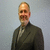## Data Center power & heat calculation formulaTags:
Data center cooling
Data Center power management
DataCenter
What is the formula to calculate power consumption in KWH and the heat dissipation in KWH or BTU?

Thanks. We'll let you know when a new response is added.

You can use this formula to calculate (really estimate) KWh consumption:

Wattage × Hours Used Per Day ÷ 1000 = Daily Kilowatt-hour (kWh) consumption

You can also use this formula to calculate (again really estimate) the heat output of electronics:
Watts * 3.412 = Btu/hour (Note: some references out on the web use 3.413 instead of 3.412.)

## Discuss This Question: 1 Reply

There was an error processing your information. Please try again later.
Thanks. We'll let you know when a new response is added.
Send me notifications when members answer or reply to this question.
•To calculate the cooling requirements for electronics you really only need to use the total KW or BTU's as the electronics run all the time and thus the electronics are producing heat all the time and cooling is required all the time. If you turn the eg; servers off then the cooling can be turned off or reduced and most cooling systems will automatically reduce their capacity. For calculation reasons it is best to assume that 100% of the power in to the electronics is converted to heat. ( the only difference if the very small amount of output electron flow) Thus if you have 100kw of power use you need 100kw of cooling plus redundancy to allow for cooling equipment failures and servicing. You may also need to add additional cooling capacity to allow for the electronic equipment lay out or the computer room infrastructure design. Eg; bad cooling delivery or overloaded areas. David Mc
report